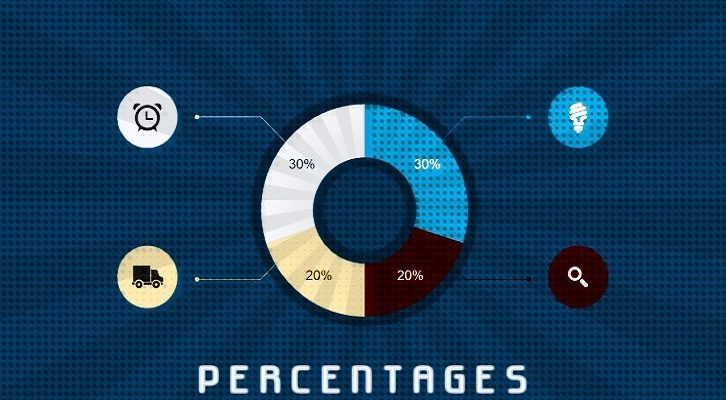# CAT – Percents

Question:

P is x% more than Q. Q is (x – 10)% less than R. If P > R, what is the range of values x can take?
A.  10% to 28%
B.  10% to 25%
C.  10% to 37%
D.  10% to 43%
Explanation:
P = Q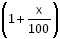Q = R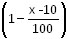R =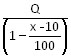P > R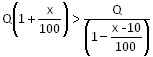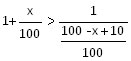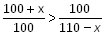(100 + x) (110 – x) > 100 x 100
11,000 + 110x – 100x – x2> 10000
1000 + 10x – x2> 0
x2 – 10x – 1000 < 0
x2 – 10x + 25 < 1000 + 25
(x – 5)2 < 1025
x – 5 < 32
x < 37
x could range from 10% to 37%